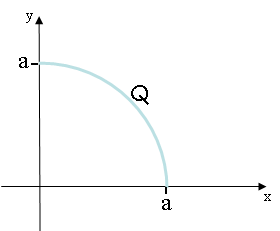# Problem: A total charge Q = 3.6 μC is distributed uniformly over a quarter circle arc of radius a = 8.8 cm as shown.a) What is λ the linear charge density along the arc?b )What is Ex, the value of the x-component of the electric field at the origin (x,y) = (0,0)?c) What is Ey, the value of the y-component of the electric field at the origin (x,y) = (0,0)?d) How does the magnitude of the electric field at the origin for the quarter-circle arc you have just calculated comnpare to the electric field at the origin produced by a point charge Q = 3.6 μC located a distance a = 8.8 cm from the origin along a 45o line as shown in the figure?    A. The magnitude of the field from the point charge is less than that from the quarter-arc of charge.    B. The magnitude of the field from the point charge is equal to that from the quarter-arc of charge    C. The magnitude of the field from the point charge is greater than that from the quarter-arc of charge

###### FREE Expert Solution

Linear charge density:

$\overline{){\mathbf{\lambda }}{\mathbf{=}}\frac{\mathbf{Q}}{\mathbf{L}}}$

a)

L = (π/2)a

Substituting to the equation for the linear charge density:

$\mathbit{\lambda }\mathbf{=}\frac{\mathbf{3}\mathbf{.}\mathbf{6}\mathbf{×}{\mathbf{10}}^{\mathbf{-}\mathbf{6}}}{\mathbf{\left(}\frac{\mathbf{\pi }}{\mathbf{2}}\mathbf{\right)}\mathbf{\left(}\mathbf{8}\mathbf{.}\mathbf{8}\mathbf{×}{\mathbf{10}}^{\mathbf{-}\mathbf{2}}\mathbf{\right)}}$

86% (48 ratings)###### Problem Details

A total charge Q = 3.6 μC is distributed uniformly over a quarter circle arc of radius a = 8.8 cm as shown.a) What is λ the linear charge density along the arc?

b )What is Ex, the value of the x-component of the electric field at the origin (x,y) = (0,0)?

c) What is Ey, the value of the y-component of the electric field at the origin (x,y) = (0,0)?d) How does the magnitude of the electric field at the origin for the quarter-circle arc you have just calculated comnpare to the electric field at the origin produced by a point charge Q = 3.6 μC located a distance a = 8.8 cm from the origin along a 45o line as shown in the figure?

A. The magnitude of the field from the point charge is less than that from the quarter-arc of charge.
B. The magnitude of the field from the point charge is equal to that from the quarter-arc of charge
C. The magnitude of the field from the point charge is greater than that from the quarter-arc of charge